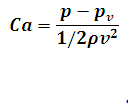# Cavitation Number Calculator

This Calctown Calculator calculates the cavitation number in fluid flow calculations used to characterize potential of the flow to cavitate.

Pa
Pa
kg/m3
m/s

#### Resultwhere

Ca = cavitation number

p = local or reference pressure

pv = vapor pressure of fluid

ρ = density of fluid

v = velocity of the fluid